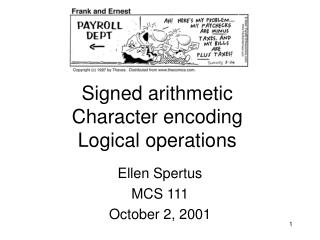Download PresentationSigned arithmetic Character encoding Logical operations

# Signed arithmetic Character encoding Logical operations - PowerPoint PPT PresentationDownload Presentation## Signed arithmetic Character encoding Logical operations

- - - - - - - - - - - - - - - - - - - - - - - - - - - E N D - - - - - - - - - - - - - - - - - - - - - - - - - - -
##### Presentation Transcript

1. Signed arithmeticCharacter encodingLogical operations Ellen Spertus MCS 111 October 2, 2001

2. Today • Review: unsigned numbers • Signed numbers • MIPS instructions for signed and unsigned instructions • Character encoding • Logical operations

3. Unsigned binary arithmetic • Biggest number? • Smallest number?

4. Signed binary arithmetic (two’s complement) • Sign bit • Biggest number? • Smallest number?

5. Signed binary to decimal

6. Shortcut • To negate a number • Invert each bit • Add 1 • Practice • 1001  0110 0111 (710), so the original was -7 • 1111 • 1000

7. Relative sizes • Which 4-bit number is bigger: • 1000 or 0111? • Signed comparison • Unsigned comparison

8. The number 710 0111 00000111 0000000000000111 The number -110 1111 11111111 1111111111111111 Sign extension Extend the sign bit all the way to the left.

9. A closer look at load-byte (lb) • load byte signed (lb) • Treat the byte as a signed number • Sign extend it to word length • lb \$t0, 400(\$zero) • load byte unsigned (lbu) • Treat the byte as an unsigned number • Zero extend it to word length • lbu \$t0, 400(\$zero)

10. Signed numbers set less than (slt) set less than immediate (slti) load byte (lb) Unsigned numbers set less than unsigned (sltu) set less than immediate unsigned (sltiu) load byte unsigned (lbu) MIPS instructions a0 = 11111111111111111111111111111111two a1 = 00000000000000000000000000000000two slt \$t0, \$a0, \$a1 # signed comparison sltu \$t1, \$a0, \$a1 # unsigned comparison

11. How do we add signed numbers? Just like we always have! • signed unsigned • -128 64 32 16 8 4 2 1 • 1 0 0 0 0 0 0 1 • 0 0 0 0 0 0 1 1

12. What about overflow? • signed unsigned • -128 64 32 16 8 4 2 1 • 0 1 1 1 1 1 1 1 • 0 1 1 1 1 1 1 1

13. Definition of overflow • When a carry bit flows into the sign bit • This can only happen with signed arithmetic • Machine raises an exception

14. Signed numbers set less than (slt) set less than immediate (slti) load byte (lb) add add immediate (addi) sub subtract immediate (subi) Unsigned numbers set less than unsigned (sltu) set less than immediate unsigned (sltiu) load byte unsigned (lbu) add unsigned (addu) add immediate unsigned (addiu) subtract unsigned (subu) subtract immediate unsigned (subiu) MIPS instructions (2)

15. Pseudo-instructions • Definition: Instructions converted by the assembler into real machine instructions • Examples: • Load immediate: li \$r1, 5 is replaced by: • Subtract immediate: subi \$r1, \$r1, 5 is replaced by:

16. Differences between signed and unsigned instructions • Sign extension vs. Zero extension • lb/lbu • Whether to signal overflow/underflow • add/addu, addi/addiu, sub/subu • How to compare numbers • slt/sltu, slti/sltiu

17. Remainder of today • Character encoding • Logical operations • Bitwise operations • Shifting

18. Character encoding • Problem: How to represent characters • Letters • Numbers • Punctuation • Control characters • Etc. • How many are there?

19. ASCII • American Standard Code for Information Interchange • 128 possibilities (7 bits) • Ctrl-a 000 00012 = 0x01 (hexadecimal) • A 100 00012 = • a 110 00012 =

20. What’s missing?

21. Alternatives • Extended ASCII (8 bits) • Not standardized • Unicode (multiple bytes) • Standardized • International character sets

22. Bitwise operations • Perform the operation on each bit position • Practice • Initial values • \$t0 = ..00000111 • \$t1 = ..00000001 • Problems • not \$t2, \$t0 • and \$t2, \$t0, \$t1 • or \$t2, \$t0, \$t1

23. Shifting • Assume \$t0 = 0..00001100 • Shift left logical (sll) • sll \$t1, \$t0, 2 • result: • Shift right logical (srl) • srl \$t1, \$t0, 2 • result: • Note: Zeroes are shifted in